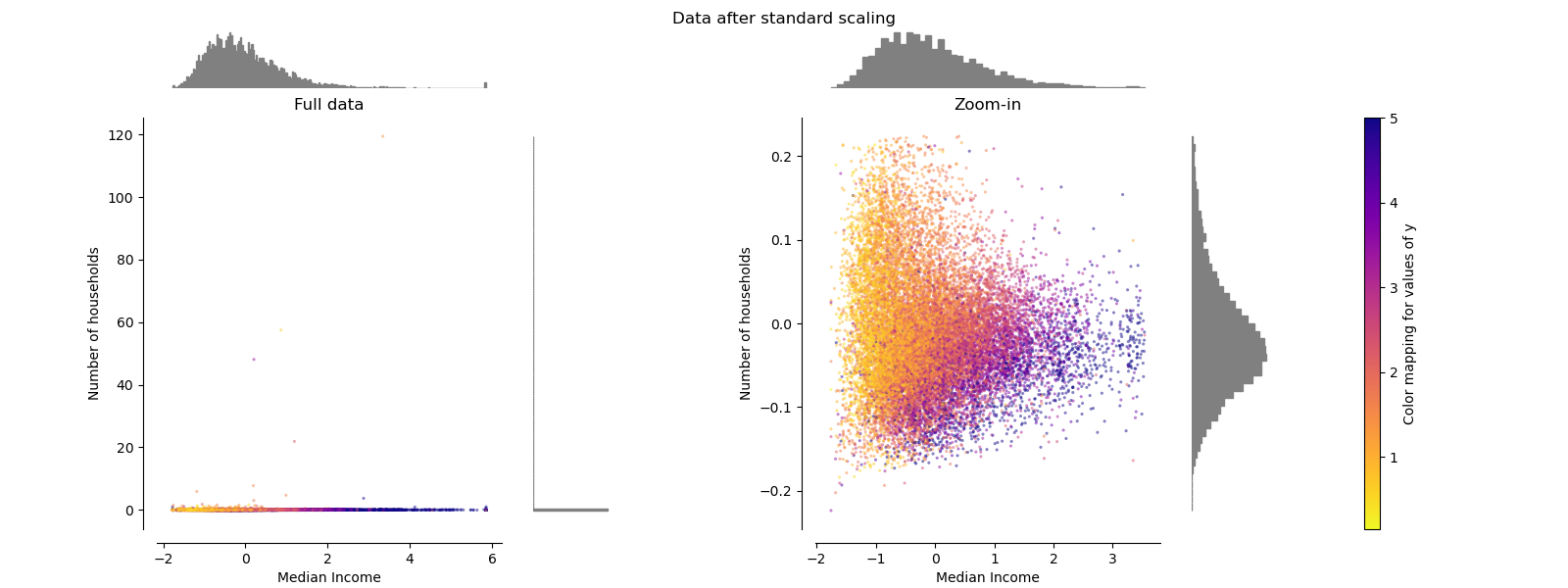# sklearn.preprocessing.RobustScaler¶

class sklearn.preprocessing.RobustScaler(*, with_centering=True, with_scaling=True, quantile_range=(25.0, 75.0), copy=True)

[源码]

with_centering boolean, True by default

with_scaling boolean, True by default

quantile_range tuple (q_min, q_max), 0.0 < q_min < q_max < 100.0

copy boolean, optional, default is True

center_ array of floats

scale_ array of floats

0.17版中的新功能：scale_属性。

robust_scale

sklearn.decomposition.PCA

https://en.wikipedia.org/wiki/Median https://en.wikipedia.org/wiki/Interquartile_range

>>> from sklearn.preprocessing import RobustScaler>>> X = [[ 1., -2.,  2.],...      [ -2.,  1.,  3.],...      [ 4.,  1., -2.]]>>> transformer = RobustScaler().fit(X)>>> transformerRobustScaler()>>> transformer.transform(X)array([[ 0. , -2. ,  0. ],       [-1. ,  0. ,  0.4],       [ 1. ,  0. , -1.6]])

fit(X[, y]) 计算要用于缩放的中位数和分位数。
fit_transform(X[, y]) 拟合数据，然后对其进行转换。
get_params([deep]) 获取此估计量的参数。
inverse_transform(X) 将数据按比例缩小到原始表示形式
set_params(**params) 设置此估算器的参数。
transform(X) 居中并缩放数据。
__init__(*, with_centering=True, with_scaling=True, quantile_range=(25.0, 75.0), copy=True)

[源码]

fit(X, y=None)

[源码]

X array-like, shape [n_samples, n_features]

fit_transform(X, y=None, **fit_params)

[源码]

X {array-like, sparse matrix, dataframe} of shape (n_samples, n_features)
y ndarray of shape (n_samples,), default=None

**fit_params dict

X_new ndarray array of shape (n_samples, n_features_new)

get_params(deep=True)

[源码]

deep bool, default=True

params mapping of string to any

inverse_transform(X)

[源码]

X **array-like **

set_params(**params)

**params dict

self object

transform(X)

[源码]

X {array-like, sparse matrix}

## sklearn.preprocessing.RobustScaler使用示例¶